# Logical conjunction

﻿
Logical conjunctionVenn diagram of$~A \and B$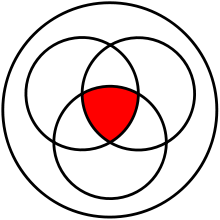Venn diagram of$~A \and B \and C$

In logic and mathematics, a two-place logical operator and, also known as logical conjunction, results in true if both of its operands are true, otherwise the value of false.

The analogue of conjunction for a (possibly infinite) family of statements is universal quantification, which is part of predicate logic.

## Notation

And is usually expressed with the prefix operator K, or an infix operator. In mathematics and logic, the infix operator is usually ; in electronics$\cdot$; and in programming languages, & or and. Some programming languages have a related control structure, the short-circuit and, written &&, and then, etc.

## Definition

Logical conjunction is an operation on two logical values, typically the values of two propositions, that produces a value of true if and only if both of its operands are true.

### Truth table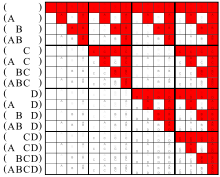Conjunctions of the arguments on the left
The true bits form a Sierpinski triangle

The truth table of$~A \and B$:

 INPUT OUTPUT A B A AND B 0 0 0 0 1 0 1 0 0 1 1 1

## Introduction and elimination rules

As a rule of inference, conjunction introduction is a classically valid, simple argument form. The argument form has two premises, A and B. Intuitively, it permits the inference of their conjunction.

A,
B.
Therefore, A and B.

or in logical operator notation:

A,
B$\vdash A \and B$

Here is an example of an argument that fits the form conjunction introduction:

Bob likes apples.
Bob likes oranges.
Therefore, Bob likes apples and oranges.

Conjunction elimination is another classically valid, simple argument form. Intuitively, it permits the inference from any conjunction of either element of that conjunction.

A and B.
Therefore, A.

...or alternately,

A and B.
Therefore, B.

In logical operator notation:$A \and B$$\vdash A$

...or alternately,$A \and B$$\vdash B$

## Properties

commutativity: yes$A \and B$$\Leftrightarrow$$B \and A$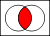$\Leftrightarrow$associativity: yes$~A$$~~~\and~~~$$(B \and C)$$\Leftrightarrow$$(A \and B)$$~~~\and~~~$$~C$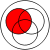$~~~\and~~~$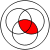$\Leftrightarrow$$\Leftrightarrow$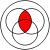$~~~\and~~~$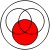distributivity: with various operations, especially with or$~A$$\and$$(B \or C)$$\Leftrightarrow$$(A \and B)$$\or$$(A \and C)$$\and$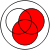$\Leftrightarrow$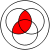$\Leftrightarrow$$\or$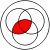idempotency: yes$~A~$$~\and~$$~A~$$\Leftrightarrow$$A~$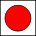$~\and~$$\Leftrightarrow$monotonicity: yes$A \rightarrow B$$\Rightarrow$$(A \and C)$$\rightarrow$$(B \and C)$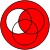$\Rightarrow$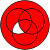$\Leftrightarrow$$\rightarrow$truth-preserving: yes
When all inputs are true, the output is true.$A \and B$$\Rightarrow$$A \and B$$\Rightarrow$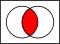(to be tested)

falsehood-preserving: yes
When all inputs are false, the output is false.$A \and B$$\Rightarrow$$A \or B$$\Rightarrow$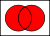(to be tested)

Walsh spectrum: (1,-1,-1,1)

Nonlinearity: 1 (the function is bent)

If using binary values for true (1) and false (0), then logical conjunction works exactly like normal arithmetic multiplication.

## Applications in computer engineering

In high-level computer programming and digital electronics, logical conjunction is commonly represented by an infix operator, usually as a keyword such as "AND", an algebraic multiplication, or the ampersand symbol "&". Many languages also provide short-circuit control structures corresponding to logical conjunction.

Logical conjunction is often used for bitwise operations, where 0 corresponds to false and 1 to true:

• 0 AND 0  =  0,
• 0 AND 1  =  0,
• 1 AND 0  =  0,
• 1 AND 1  =  1.

The operation can also be applied to two binary words viewed as bitstrings of equal length, by taking the bitwise AND of each pair of bits at corresponding positions. For example:

• 11000110 AND 10100011  =  10000010.

This can be used to select part of a bitstring using a bit mask. For example, 10011101 AND 00001000  =  00001000 extracts the fifth bit of an 8-bit bitstring.

In computer networking, bit masks are used to derive the network address of a subnet within an existing network from a given IP address, by ANDing the IP address and the subnet mask.

Logical conjunction "AND" is also used in SQL operations to form database queries.

The Curry-Howard correspondence relates logical conjunction to product types.

## Set-theoretic intersection

The intersection used in set theory is defined in terms of a logical conjunction: xAB if and only if (xA) ∧ (xB). Because of this, set-theoretic intersection shares several properties with logical conjunction, such as associativity, commutativity, and idempotence.

## Natural language

The logical conjunction and in logic is related to, but not the same as, the grammatical conjunction and in natural languages.

English "and" has properties not captured by logical conjunction. For example, "and" sometimes implies order. For example, "They got married and had a child" in common discourse means that the marriage came before the child. The word "and" can also imply a partition of a thing into parts, as "The American flag is red, white, and blue." Here it is not meant that the flag is at once red, white, and blue, but rather that it has a part of each color.

Wikimedia Foundation. 2010.

### Look at other dictionaries:

• Conjunction — can refer to: Conjunction (astronomy and astrology), an astronomical phenomenon Astrological aspect, an aspect in horoscopic astrology Conjunction (grammar), a part of speech Conjunctive mood (grammar), same as subjunctive mood Logical… …   Wikipedia

• Logical disjunction — Disjunction redirects here. For separation of chromosomes, see Meiosis. For disjunctions in distribution, see Disjunct distribution. Venn diagram of the logical disjunction of A and B …   Wikipedia

• Conjunction (grammar) — But redirects here. For other uses, see BUT (disambiguation). In grammar, a conjunction (abbreviated conj or cnj) is a part of speech that connects two words, sentences, phrases or clauses together. A discourse connective is a conjunction joining …   Wikipedia

• Logical truth — is one of the most fundamental concepts in logic, and there are different theories on its nature. A logical truth is a statement which is true and remains true under all reinterpretations of its components other than its logical constants. It is… …   Wikipedia

• Logical connective — This article is about connectives in classical logic. For connectors in natural languages, see discourse connective. For connectives and operators in other logics, see logical constant. For other logical symbols, see table of logic symbols. In… …   Wikipedia

• Logical implication — In logic and mathematics, logical implication is a logical relation that holds between a set T of formulae and a formula B when every model (or interpretation or valuation) of T is also a model of B . In symbols,# T models B, # T Rightarrow B # T …   Wikipedia

• Conjunction fallacy — The conjunction fallacy is a logical fallacy that occurs when it is assumed that specific conditions are more probable than a single general one. The most often cited example of this fallacy originated with Amos Tversky and Daniel Kahneman:… …   Wikipedia

• Logical symbols — Reading logical symbolism frightens many people more than it should. The very term symbolic logic sounds terrifying, and the presence of even a small amount of symbolism may deter many readers from otherwise perfectly intelligible texts. The… …   Philosophy dictionary

• Logical NOR — This article is about NOR in the logical sense. For the electronic gate, see NOR gate. For other uses, see Nor. Venn diagram of In boolean logic, logical nor or joint denial is a truth functional operator which produces a result that is the… …   Wikipedia

• Logical biconditional — In logic and mathematics, the logical biconditional (sometimes known as the material biconditional) is the logical connective of two statements asserting p if and only if q , where q is a hypothesis (or antecedent) and p is a conclusion (or… …   Wikipedia

We are using cookies for the best presentation of our site. Continuing to use this site, you agree with this.